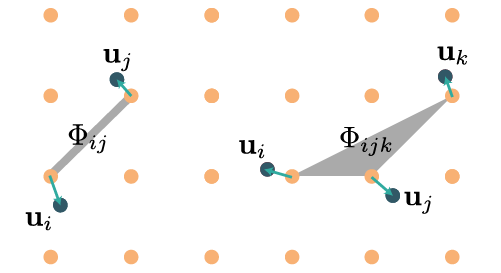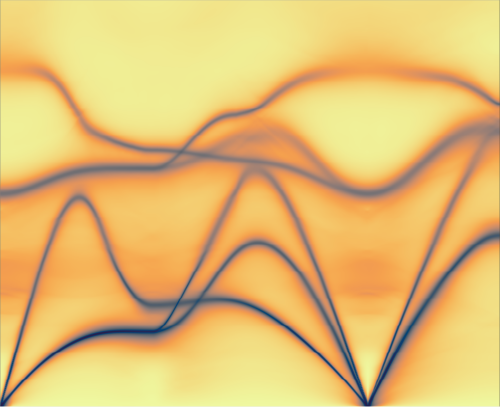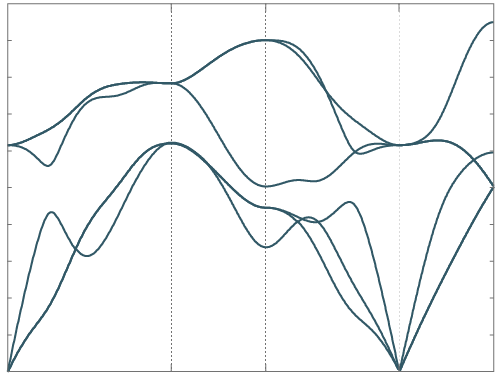### atomic distribution

Calculates properties of the atomic distribution from molecular dynamics, such as mean square displacement, pair distribution function, vector distribution functions and probability densities. Useful for analysing simulations close to instabilities/phase transitions to have some idea where the atoms are.

### canonical configuration

Use forceconstants or a Debye temperature to generate uncorrelated supercell configurations emulating a canonical ensemble. These configurations can be used to either start ensemble runs of molecular dynamics with negligible equilibration time, or be used to directly sample phase space.

### crystal structure info

This code serves as a diagnostic tool to check that symmetry heuristics are working as they should. The code prints which Bravais lattice was identified, which high symmetry points in the BZ are inequivalent, and so on. The Brillouin zone and its irreducible wedges are printed to files as polyhedra for manual inspection, and the symmetry operations of the lattice can be printed.

### extract forceconstantsThe main algorithm of the TDEP method. Starting with a symmetry analysis, this code finds the irreducible representation of interatomic forceconstants and extracts them from position and force data.

### generate structure

Builds supercells, diagonal and non-diagonal. Also has the capability to find the optimal supercells for a given lattice, very handy when you have complicated structures.

### lineshapeCalculate the frequency-dependent self-energy and phonon spectral function from many-body perturbation theory.

### phonon dispersion relationsCalculate phonon dispersions and related quantities. Per default, only the dispersions along a default path will be calculated. Options are available for calculating mode gruneisen parameters, phonon density of states projected in a variety of ways, thermodynamic quantities and pure data dumps.

### samples from md

Choose representative uncorrelated samples from an MD simulation. The samples are chosen to be approximately evenly spaced, and reproduce the average potential energy, average kinetic energy have the same standard deviation of potential and kinetic energy.

### thermal conductivity

Calculates the lattice thermal conductivity from the iterative solution of the phonon Boltzmann equation. In addition, cumulative plots and raw data dumps of intermediate values are available.